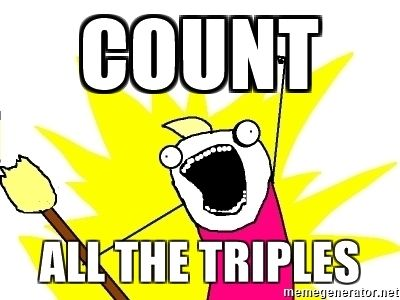시간 제한 메모리 제한 제출 정답 맞은 사람 정답 비율
7 초 512 MB 6 3 3 50.000%

## 문제

You are given a tree, i.e. a connected undirected graph with no cycles. For every two vertices $x, y$ let $d(x, y)$ denote the length (i.e. the number of edges) of the unique simple path between $x$ and $y$. Count all the (unordered) triples $\{x,y,z\}$ such that $d(x,y) = d(y,z) = d(z,x) > 0$.## 입력

The first line of input contains the number of test cases $z$ ($1 \leq z \leq 20$). The descriptions of the test cases follow.

The first line of every test case contains the number of vertices $n$ ($3 \leq n \leq 100\,000$). Each of the next $n - 1$ lines contains two integers $a, b$ ($1 \leq a, b \leq n$), denoting that there is an edge between vertices $a$ and $b$.

## 출력

For each test case output one integer: the number of triples in question.

## 예제 입력 1

2
4
1 2
1 3
1 4
8
1 2
1 3
1 4
2 5
2 6
3 7
4 8


## 예제 출력 1

1
4